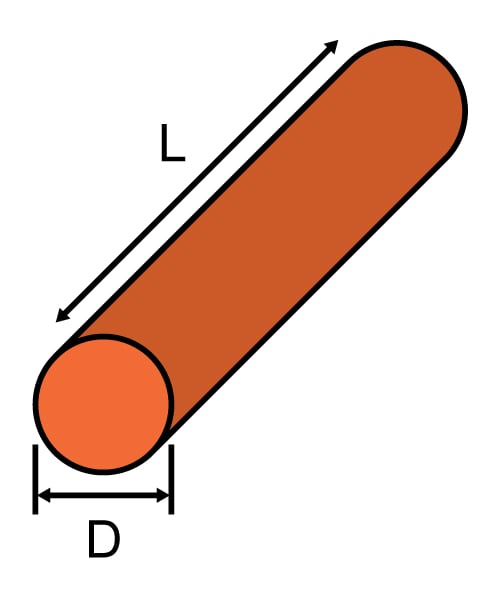# Wire Self Inductance Calculator

## This calculator will help compute the inductance of a length of wire.

nH

### Overview

Even a simple length of wire can exhibit inductance, or rather, self-inductance. This tool is designed to calculate the inductance of a length of wire given its diameter and length. Note that self-inductance of a wire is often not measured since it is only a part of a circuit's loop.

To use this calculator, just enter the diameter and length of the wire. You can select the unit of measurement for the diameter and the length. The output inductance is presented in nano henries (10-9 H).### Equation

$$L_{wire}=2l\left \{ \ln \left [ \left ( \frac{2L}{D} \right )\left ( 1+\sqrt{1+\left ( \frac{D}{2L} \right )^{2}} \right ) \right ]-\sqrt{1+\left ( \frac{D}{2L} \right )^{2}}+\frac{\mu }{4}+\left ( \frac{D}{2L} \right ) \right \}$$

Where:

$$L_{wire}$$ = inductance of a straight wire in henries (H)

$$D$$ = diameter of the wire in cm

$$L$$ = length of the wire cm

$$\mu$$ = permeability

Note: The equation above is from “Inductance Calculations” by F. W. Grover

### Applications

A wire can be found in any circuit, and thus, it is important to know how much inductance it is contributing. Any inductor at a specific frequency can attenuate or filter out a signal. The self-inductance of a straight wire may only be defined as only a partial inductance and so cannot be measured directly because of the absence of a return path. In contrast, a loop inductance can be measured. Therefore, the inductance of a straight wire can only be calculated or determined indirectly from measurements of the inductance of a loop of wire.•JSalmans September 20, 2021